# Analytic capacity

(diff) ← Older revision | Latest revision (diff) | Newer revision → (diff)

analytic measure, Ahlfors analytic measure

A set function in the plane, introduced by L. Ahlfors , which is an analogue of the logarithmic capacity, and which is suited for the characterization of sets of removable singularities of bounded analytic functions. Letbe a bounded closed set in the plane, let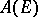be the set of functions which are analytic outside, vanish at infinity and are bounded everywhere outside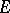by the constant 1. Letas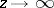. The number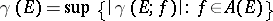, is called the analytic capacity of the set. The analytic capacity of an arbitrary set is usually defined as the supremum of the analytic capacities of its bounded closed subsets.

Ifis a bounded closed set, then a necessary and sufficient condition in order that each function which is analytic and bounded outsidebe extendable to, is that the analytic capacity ofbe zero (the Ahlfors theorem).

There also exist several concepts related to analytic capacity which are suited to the metrics of various other spaces of analytic functions (see, for example,  and ).

The concept of analytic capacity proved to be well suited to certain problems in approximation theory, where the solution of a number of fundamental problems is formulated in terms of analytic capacity. Thus, , any continuous function on a bounded closed setin the plane can be uniformly approximated by rational functions to any desired degree of accuracy, if and only if the equalityholds for any disc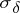of radius.

How to Cite This Entry:
Analytic capacity. Encyclopedia of Mathematics. URL: http://encyclopediaofmath.org/index.php?title=Analytic_capacity&oldid=11845
This article was adapted from an original article by A.G. Vitushkin (originator), which appeared in Encyclopedia of Mathematics - ISBN 1402006098. See original article